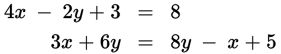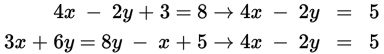# SAT Math Multiple Choice Question 799: Answer and Explanation

### Test Information

Question: 7994. How many solutions does the system of equations shown above have?

• A. 0
• B. 1
• C. 2
• D. Infinitely many

Explanation:

D

Difficulty: Easy

Category: Heart of Algebra / Systems of Linear Equations

Strategic Advice: When a question about a system of equations asks for the number of solutions, rather than the actual solution, don't worry about finding the value of x and y right away-you might not even need them to answer the question. Just simplify the two equations as much as possible, and then compare them.

Getting to the Answer: For each equation, combine like terms and write the results in standard form (Ax + By = C):The equations are identical (dependent) and therefore represent the same line, which means the system has infinitely many solutions, making (D) correct.